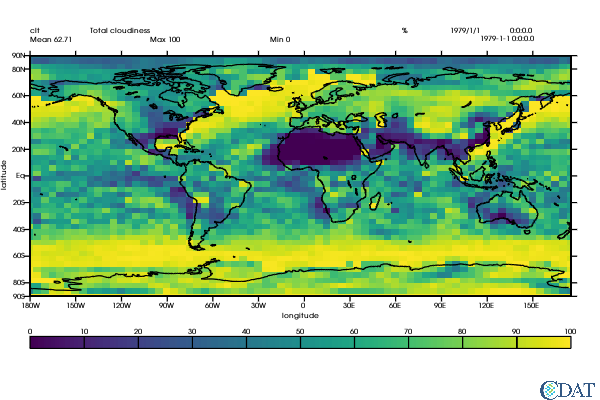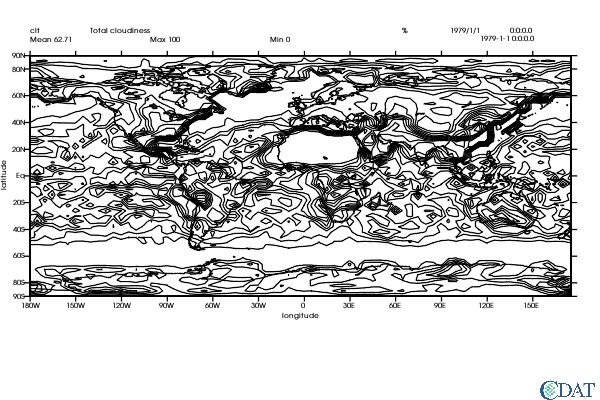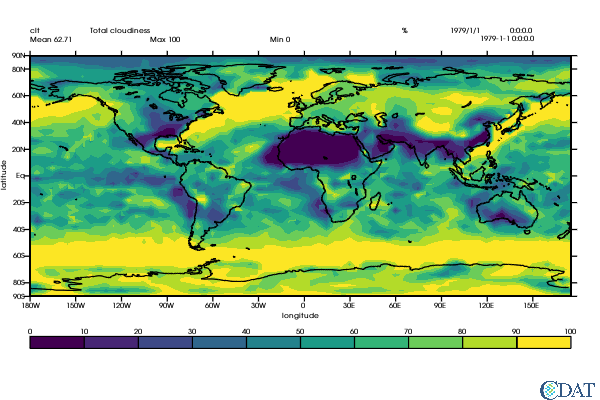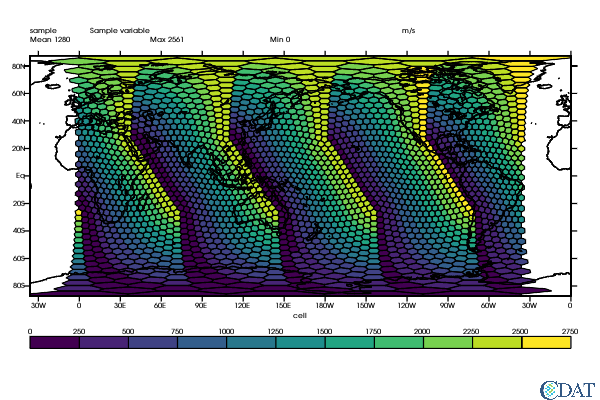# Visualization Control System Principles¶

VCS Allows scientists to produce highly customized plots. Everything can be precisely and logically controlled.

To download this Jupyter Notebook, right click on the link and choose "Download Linked File As..." or "Save Link as...". Remember where you saved the downloaded file, which should have an .ipynb extension. (You'll need to launch the Jupyter notebook or JupyterLab instance from the location where you store the notebook file.)

In :
# VCS Object definitions
import vcs
import cdms2
import os
with cdms2.open(os.path.join(vcs.sample_data,"clt.nc")) as f:
clt = f("clt")
u = f("u")
v = f("v")
with cdms2.open(os.path.join(vcs.sample_data,"sampleCurveGrid4.nc")) as f:
curv = f("sample")
with cdms2.open(os.path.join(vcs.sample_data,"sampleGenGrid3.nc")) as f:
gen = f("sample")

x = vcs.init(geometry=(600,400),bg=True)

# Styling for the notebook
from IPython.core.display import HTML
HTML("""
<style>
.output_png {
display: table-cell;
text-align: center;
vertical-align: middle;
}
</style>
""")

Downloading: 'BlueMarble.ppm' from 'https://cdat.llnl.gov/cdat/sample_data/' in: /Users/davis278/miniconda3/envs/cdat81-mesa/share/cdat/sample_data/BlueMarble.ppm

Out:

## Introduction¶

Essentially a vcs plot can be broken down into three parts:

WHAT is plotted (e.g data and labels) HOW it is rendered (isolines, boxfill, isofill, vectors, etc...) and WHERE (the location on the page where each element is to be plotted)

## What¶

This is the scientific piece of information that the user is trying to represent for others (or oneself) to understand. It can be as raw as a simple numpy object. It is recommended to use CDMS's transient variables. CDMS transient variables contain metadata such as name, units, and geospatial information that can be used by VCS to better represent the data.

The [tutorials] section has many CDMS examples. The CDMS documentation can be found here.

## How¶

This describes the data representation. At the highest level it is a graphics method i.e boxfill, isofill, vectors, streamlines, line plot, etc., but it also contains information to further control these plot types, e.g. which colors to use, which levels, line thickness, etc.

Graphic methods also describe how axes and labels should be represented (e.g. which axis values to show and which text to use for the values. The user might want to show the -20. longitude represented as 20S or the date 2020-01-15 shown as Jan 2020.

Currently VCS supports the following graphic methods:

### Boxfill¶

Boxfill is used to represent 2-dimensional arrays, filling each array cell with a color representing its value. In the case of rectilinear grids where x and y axes can be represented by a 1-dimensional array, we use the axes bounds to determine the extent of each cell. This is especially useful if an axis is not increasing constantly (e.g. a gaussian grid or pressure levels).

In :
gm = vcs.createboxfill()
x.plot(clt, gm)

Out:### Isoline¶

An isoline is a line on a map, chart, or graph that connects points of equal value.

In :
gm = vcs.createisoline()
x.clear()
x.plot(clt,gm)

Out:### Isofill¶

The isofill graphic method is similar to isolines (and usually plotted in conjounction with it) except that the area between two consecutive isolines is filled with a color representing the range of values in this area.

In :
x.clear()
gm = vcs.createisofill()
x.plot(clt,gm)

Out:### Meshfill¶

Meshfill is very similar to boxfill, but is used to represent data on generic grids (a.k.a. native representation) based on the input data and a mesh.

In :
x.clear()
gm = x.createmeshfill()
gm.mesh = True
x.plot(gen, gm)

Out:### Streamlines¶

x.clear()